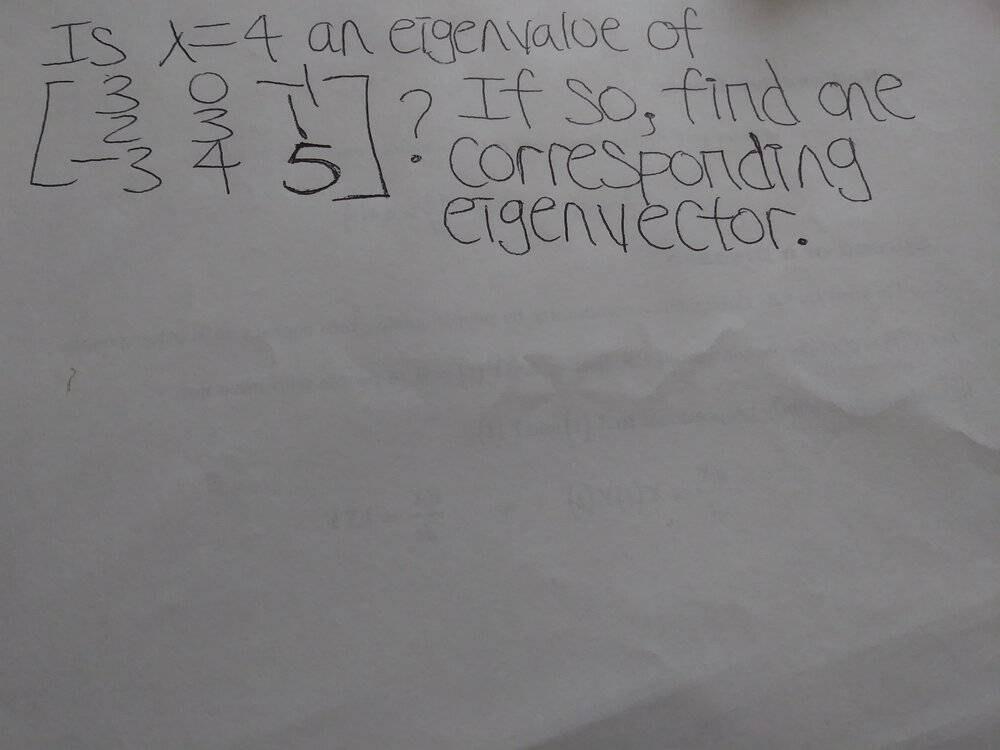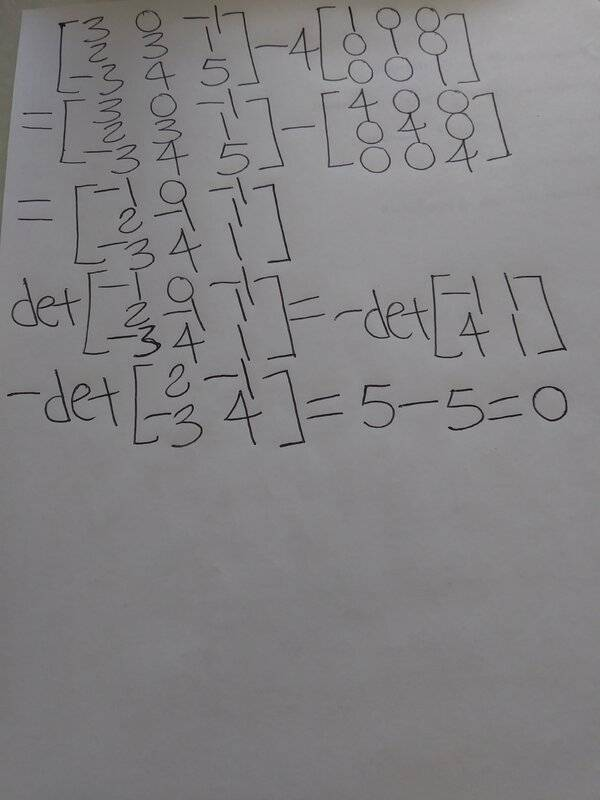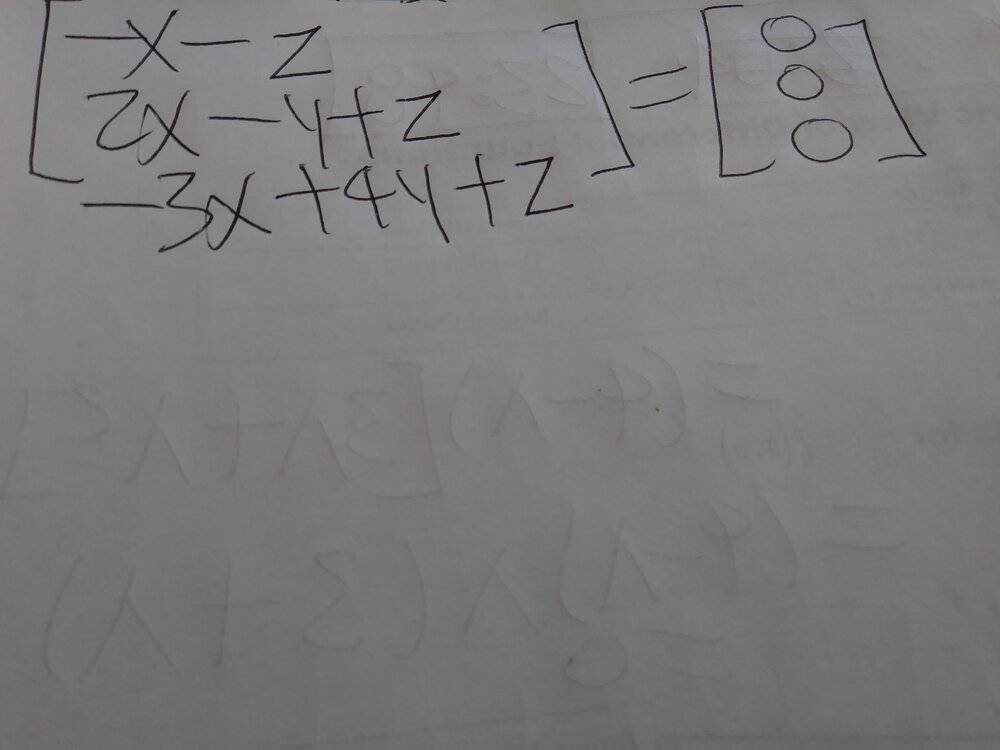# How to find one corresponding eigenvector?

Math100
Homework Statement:
Is lambda=4 an eigenvalue of the given matrix? If so, find one corresponding eigenvector.
Relevant Equations:
None.
The determinant is 0, which means that A-4I has a nullspace, and there is an eigenvector with eigenvalue 4. In the textbook, the answer says "Yes, [1, 1, -1]" for this problem. But I don't know how to find the corresponding eigenvector for this problem. Below is my work.Mentor
2022 Award
Homework Statement:: Is lambda=4 an eigenvalue of the given matrix? If so, find one corresponding eigenvector.
Relevant Equations:: None.

The determinant is 0, which means that A-4I has a nullspace, and there is an eigenvector with eigenvalue 4. In the textbook, the answer says "Yes, [1, 1, -1]" for this problem. But I don't know how to find the corresponding eigenvector for this problem. Below is my work.
You have to find a vector ##\vec{v}\neq 0## such that ##(A-4I)\vec{v}=0##. Can you solve this equation system?

•DaveE
Math100
How should I find the vector then?

Mentor
2022 Award
Solve ##\begin{bmatrix}-1&0&-1\\2&-1&1\\-3&4&1\end{bmatrix}\cdot \begin{bmatrix}x\\y\\z\end{bmatrix}=\begin{bmatrix}0\\0\\0\end{bmatrix}## for ##x,y,z##. Then you get one or more solutions which are eigenvectors to ##4##. I don't know the other eigenvalues though.

•DaveE
Gold Member
I think an easy way to remember these equations is to memorize the concept that the eigenvectors of A are the vectors that "don't change direction" when transformed by the matrix A, they only change in length. That length change is the eigenvalue for that vector. So Aν = λν. All of the equations you need (see @fresh_42) follow from this concept with some matrix algebra.

Math100
But I didn't get the answer from the book. As a result, I got x=y=z=0, the book says x=1, y=1, z=-1.Mentor
2022 Award
You get ##x=-z## from the first row. This makes ##-2z-y+z==-y-z=0## the second row and thus ##y=-z##. Do they solve the third equation, too? And if so, how do we handle ##z##?